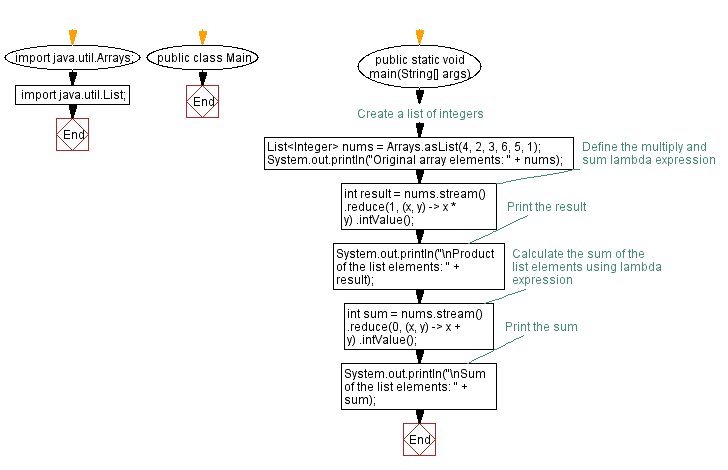# Multiply and sum elements with Lambda expression in Java

## Java Lambda Program: Exercise-12 with Solution

Write a Java program to create a lambda expression to multiply and sum all elements in a list of integers.

Sample Solution:

Java Code:

``````import java.util.Arrays;
import java.util.List;

public class Main {
public static void main(String[] args) {
// Create a list of integers
List<Integer> nums = Arrays.asList(4, 2, 3, 6, 5, 1);
System.out.println("Original array elements: " + nums);
// Define the multiply and sum lambda expression
int result = nums.stream()
.reduce(1, (x, y) -> x * y)
.intValue();

// Print the result
System.out.println("\nProduct of the list elements: " + result);

// Calculate the sum of the list elements using lambda expression
int sum = nums.stream()
.reduce(0, (x, y) -> x + y)
.intValue();

// Print the sum
System.out.println("\nSum of the list elements: " + sum);
}
}
``````

Sample Output:

```Original array elements: [4, 2, 3, 6, 5, 1]

Product of the list elements: 720

Sum of the list elements: 21
```

Explanation:

Initially the necessary classes are imported: Arrays and Lists.

In the main method, we create a list of integers named nums using the Arrays.asList() method. The list contains several integer values.

We define a lambda expression (x, y) -> x * y using the reduce() method on the stream of nums. The lambda expression takes two integers x and y as input and multiplies them using the operator.

The reduce() method applies the lambda expression to each element in the stream, performing the multiplication operation. The initial reduction value is set to 1.

We store the multiplication result in the result variable, which is an integer.

Next, we calculate the sum of all elements in the list:

We define a lambda expression (x, y) -> x + y using the reduce() method on the stream of nums. The lambda expression takes two integers x and y as input and adds them using the + operator.

The reduce() method applies the lambda expression to each element in the stream, performing the addition operation. The initial reduction value is set to 0.

We store the sum result in the sum variable, which is an integer.

Flowchart:Live Demo:

Java Code Editor:

Improve this sample solution and post your code through Disqus

Java Lambda Exercises Previous: Find maximum and minimum values with Lambda expression in Java.
Java Lambda Exercises Next: Java Program to count words in a sentence using lambda expression.

What is the difficulty level of this exercise?

Test your Programming skills with w3resource's quiz.

﻿